Question

A 2.41g sample of iron powder (Fe) reacted with 1.405 g of sulfur(S)

A 2.41g sample of iron powder (Fe) reacted with 1.405 g of sulfur(S). Calculate the empirical formula of the iron sulfide product.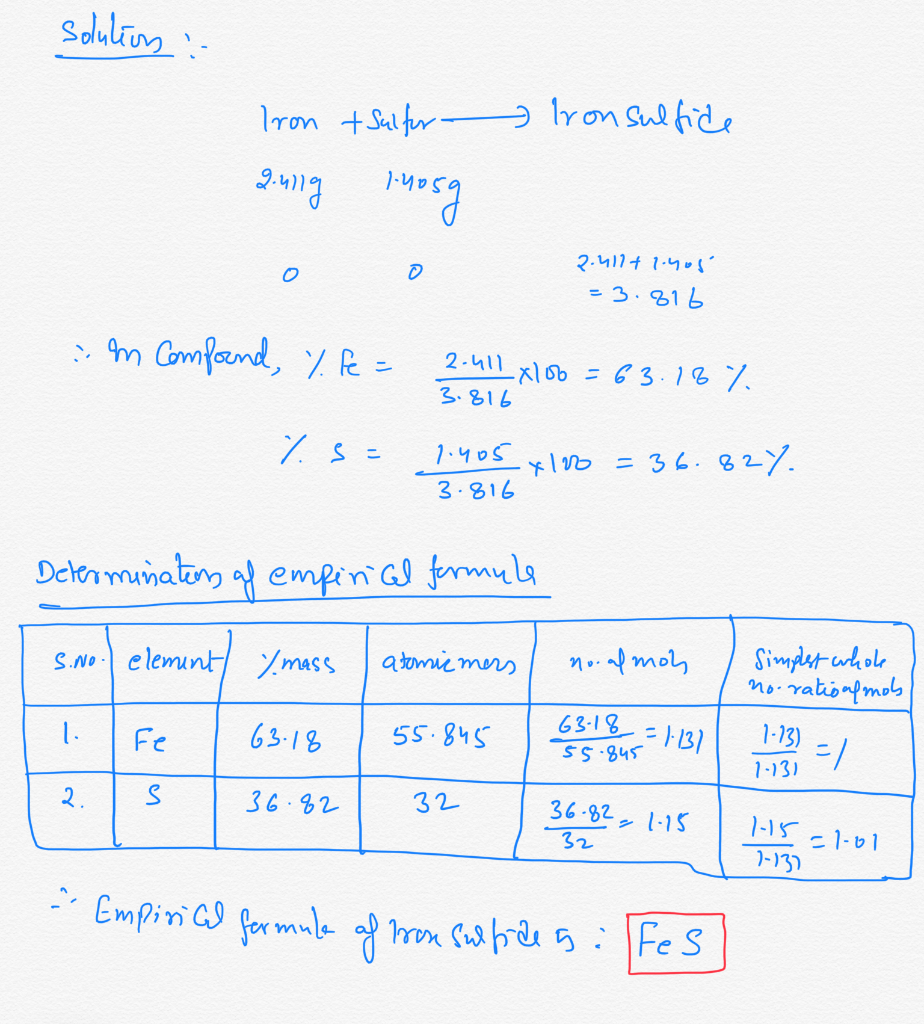Earn Coins

Coins can be redeemed for fabulous gifts.

Similar Homework Help Questions
• A 1.000-g sample of iron metal reacted with sulfur powder to give 1.574 g of prod...

5. A 1.000-g sample of iron metal reacted with sulfur powder to give 1.574 g of prod Calculate the empirical formula of the iron sulfide.6. A 1.000-g sample of iron metal reacted with sulfur powder to give 1.861 g of product Calculate the empirical formula of the iron sulfide.

• 1.167-g sample of iron powder reacted with 0.680 g of sulfur powder to give iton oduct....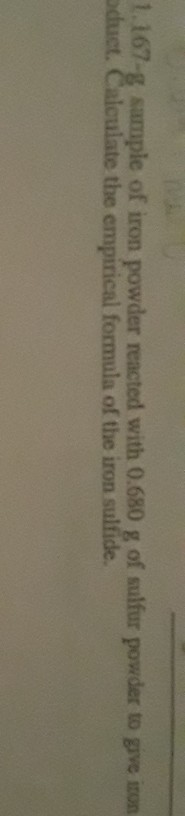1.167-g sample of iron powder reacted with 0.680 g of sulfur powder to give iton oduct. Calculate the empirical formula of the iron sulfide.

• A 0.500-g sample of chromium metal reacted with sulfur powder to give 0.963 g of product.   a) The mass of sul...

A 0.500-g sample of chromium metal reacted with sulfur powder to give 0.963 g of product.   a) The mass of sulfur that reacted is _____ b) The empirical formula of the chromium sulfide is _____

• 40.505-8 sample of cobalt metal reacted with excess sulfur powder to give 1.027 g of cobalt...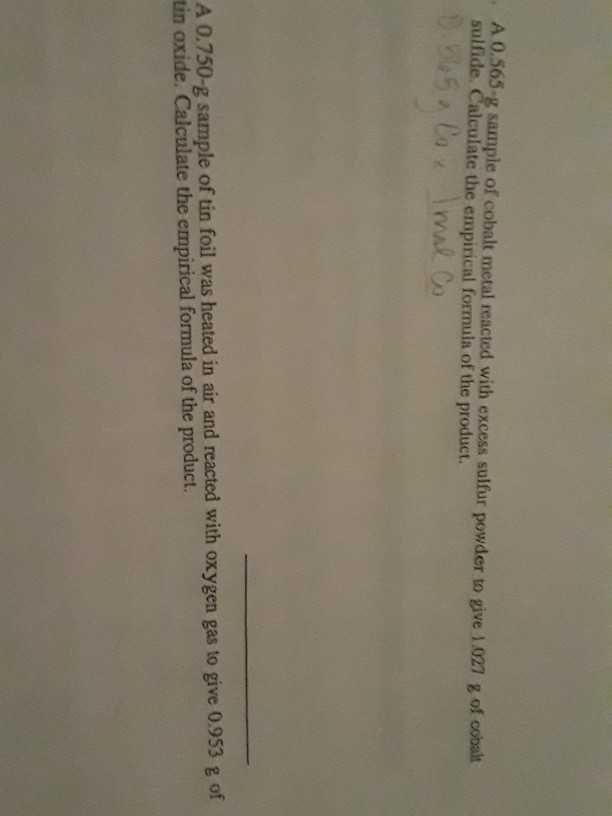40.505-8 sample of cobalt metal reacted with excess sulfur powder to give 1.027 g of cobalt sulfide. Calculate the empirical formula of the product. D. 565. Cox Imal Co A 0.750-g sample of tin foil was heated in air and reacted with oxygen gas to give 0.953 g of tin oxide. Calculate the empirical formula of the product.

• 5. A 0.565-8 sample of cobalt metal reacted with excess sulfur powder to give 1.027 g of cobalt sulfide

5. A 0.565-8 sample of cobalt metal reacted with excess sulfur powder to give 1.027 g of cobalt sulfide Calculate the empirical formula of the product. 6. A 0.750-g sample of tin foil was heated in air and reacted with oxygen gas to give 0.953 g of tin oxide. Calculate the empirical formula of the product. 7. (optional) A 1.000-g sample of red phosphorus powder was burned in air and reacted with oxygen gas to give 2.291 g of phosphorus oxide. Calculate...

• A 1.000-g sample of cobalt metal reacted with sulfur powder to give 1.548 g of product....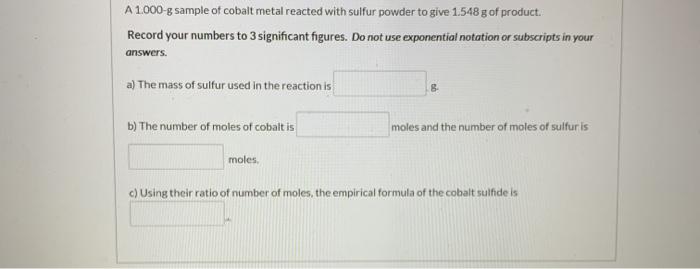A 1.000-g sample of cobalt metal reacted with sulfur powder to give 1.548 g of product. Record your numbers to 3 significant figures. Do not use exponential notation or subscripts in your answers. a) The mass of sulfur used in the reaction is b) The number of moles of cobalt is moles and the number of moles of sulfur is moles. c) Using their ratio of number of moles, the empirical formula of the cobalt sulfide is Draw the Lewis...

• 5-8 sample of cobalt metal reacted with excess salfiur powder to give 1.00 Calculate the empirical...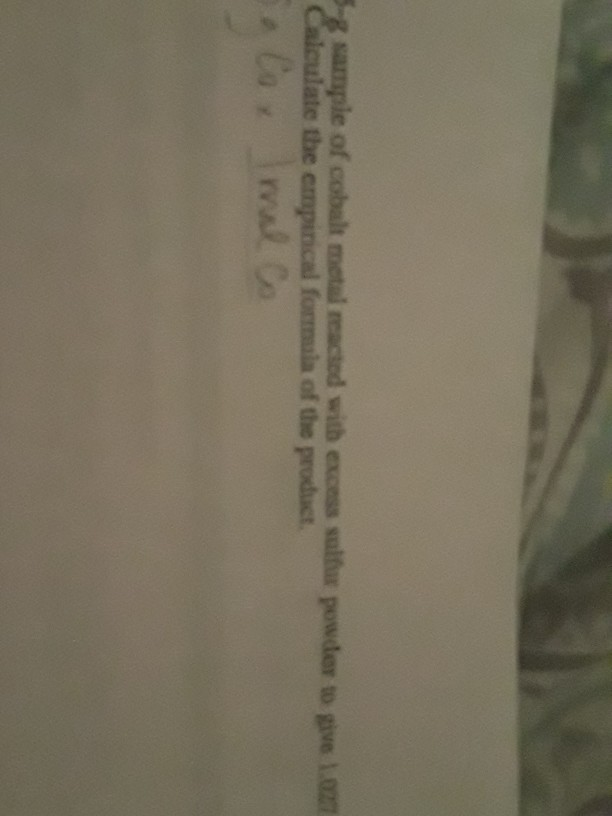5-8 sample of cobalt metal reacted with excess salfiur powder to give 1.00 Calculate the empirical formula of the product olo. Imal Co 55-g sample of cobalt metal reacted with excess sulfur powder to give 1.027 - Calculate the empirical formula of the product.

• homework

1. A 0.565 sample of cobalt metal reacted wtih excess sulfur powerd to give 1.027g of cobalt sulfide. Calculate the empirical formaula of the product.2.A. 0.750g sample of tin foil was heated in air and reacted with oxygen gas to give 0.953g of tin oxide. calculate the empirical formula of the product/3. A. 1.167g sample pf iron powder reacted with 0.680g of sulfur powder to give iron sulfide product. Calculate the empirical formula of the iron sulfide.

• HELP ASAP PLEASE When iron reacts with sulfur the product is iron (III) sulfide. Fe (s)...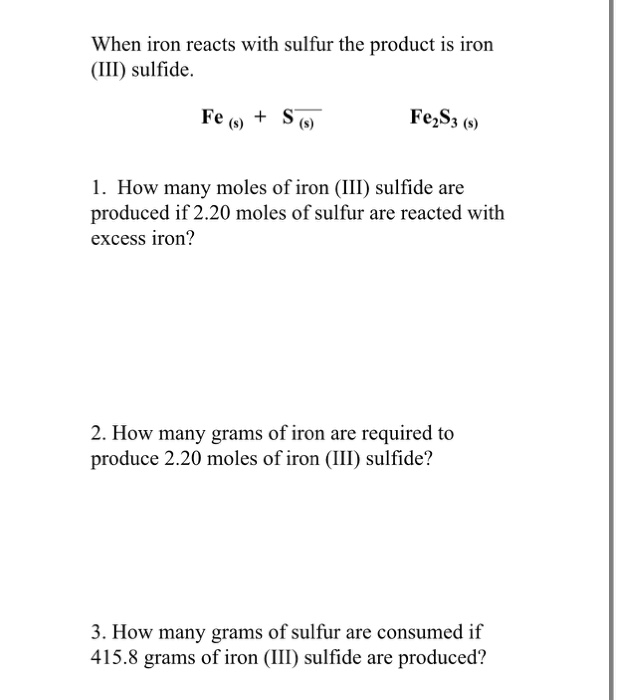HELP ASAP PLEASE When iron reacts with sulfur the product is iron (III) sulfide. Fe (s) + S (6) Fe2S3 (5) 1. How many moles of iron (III) sulfide are produced if 2.20 moles of sulfur are reacted with excess iron? 2. How many grams of iron are required to produce 2.20 moles of iron (III) sulfide? 3. How many grams of sulfur are consumed if 415.8 grams of iron (III) sulfide are produced?

• DATE NAME SECTION POSTLABORATORY ASSIGNMENT 1. Predict the empirical formula for each of the following compounds...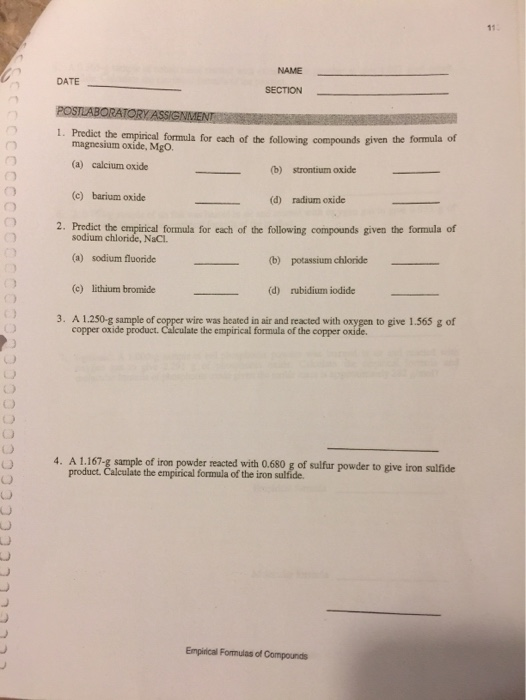DATE NAME SECTION POSTLABORATORY ASSIGNMENT 1. Predict the empirical formula for each of the following compounds given the formula of magnesium oxide, MgO. (a) calcium oxide (b) strontium oxide (c) barium oxide (d) radium oxide 2. Predict the empirical formula for each of the following compounds given the formula of sodium chloride, NaCl. (a) sodium fluoride (b) potassium chloride (c) lithium bromide (d) rubidium iodide 3. A 1.250 g sample of copper wire was heated in air and reacted with...